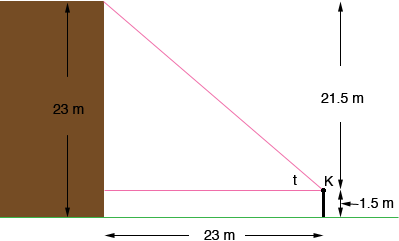Quandaries and Queries Name: chloe Who is asking: Student Level of the question: Secondary Question: Hi, I am having a bit of trouble with the following question: Karen is standing 23 metres away from the base of a 23 metre high house. Assume that Karen's eyes are 1.5 metres above ground. Find the elevation of the top of the house from Karen's eye line. Hi Chloe, I drew a diagram.K is Karen's eye, 1.5 m above the ground and t is the angle of elevation. From the diagram tan(t) = 21.5/23 Find t. Penny